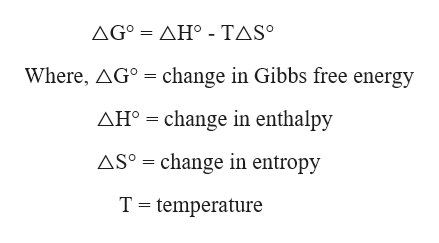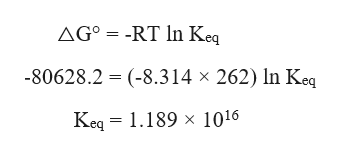# For the reaction4HCl(g) + O2(g)2H2O(g) + 2Cl2(g)H° = -114.4 kJ and S° = -128.9 J/KThe equilibrium constant for this reaction at 262.0 K is ______Assume that H° and S° are independent of temperature

Question
33 views

For the reaction

4HCl(g) + O2(g)2H2O(g) + 2Cl2(g)

H° = -114.4 kJ and S° = -128.9 J/K

The equilibrium constant for this reaction at 262.0 K is ______

Assume that H° and S° are independent of temperature

check_circle

Step 1

The Gibbs Helmholtz equation is given as,help_outlineImage TranscriptioncloseΔGΔΗ-ΤASo Where, AGo change in Gibbs free energy AH change in enthalpy change in entropy ASo T temperature fullscreen
Step 2

The Gibbs free energy can be calculated as,

Step 3

The equilibrium constant (Keq) ca...help_outlineImage TranscriptioncloseAG° = -RT In Keq -80628.2 (-8.314 x 262) In Keq Keq 1.189 x 1016 fullscreen

### Want to see the full answer?

See Solution

#### Want to see this answer and more?

Solutions are written by subject experts who are available 24/7. Questions are typically answered within 1 hour.*

See Solution
*Response times may vary by subject and question.
Tagged in

### Chemical Thermodynamics